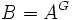# Ring is integral extension of fixed-point subring under finite automorphism group

Jump to: navigation, search

## Statement

Let$A$ be a commutative unital ring and$G$ be a finite group acting as automorphisms on$A$ (in other words,$G$ is a finite subgroup of the automorphism group of$A$). Let$B = A^G$ be the subring of$B$ comprising those elements fixed by every element of$G$. Then,$B$ is an integral extension of$A$. In fact, every element of$B$ satisfies a monic polynomial over$A$ of degree equal to the order of$G$.

## Proof

Let$x \in B$. Consider the elements$g \cdot x$ for$g \in G$. Then, all the elementary symmetric polynomials in these elements take values in$A$. Hence, we can construct a monic polynomial of degree equal to the order of$G$, with all coefficients in$A$, and whose roots are precisely the elements$g \cdot x$.